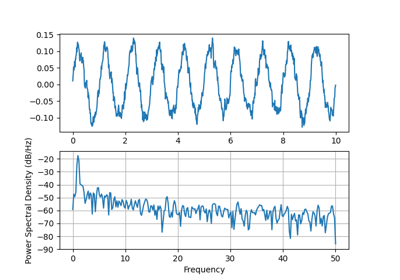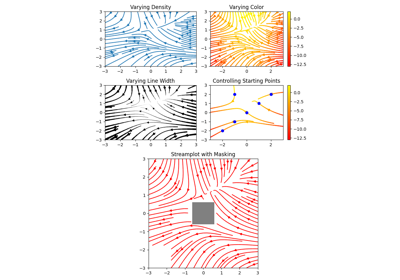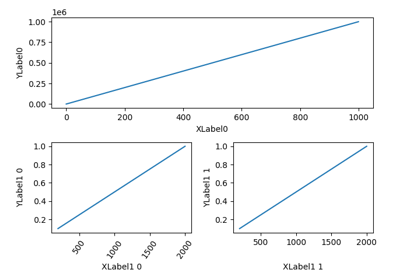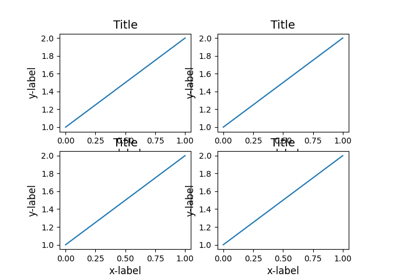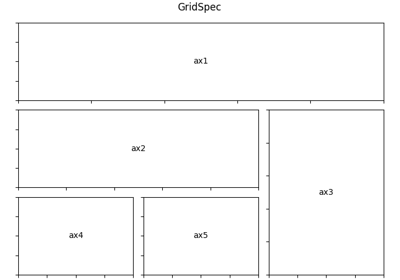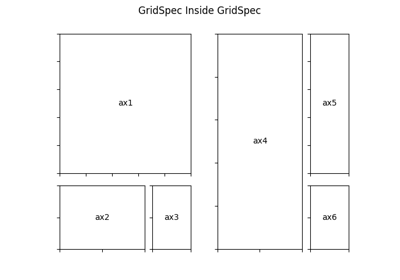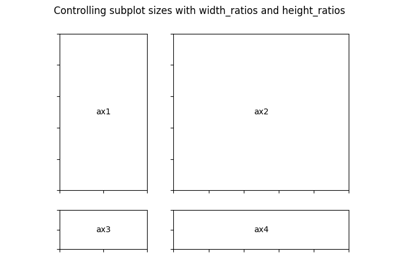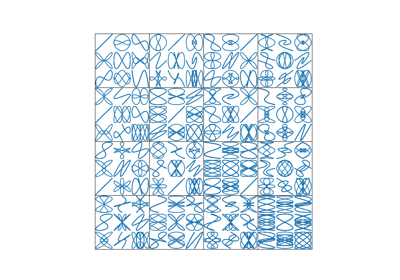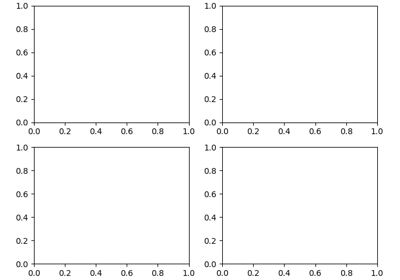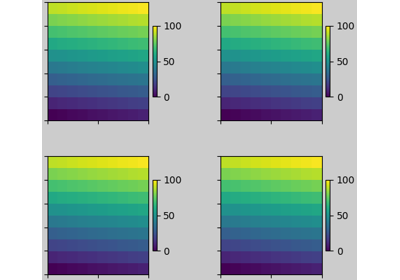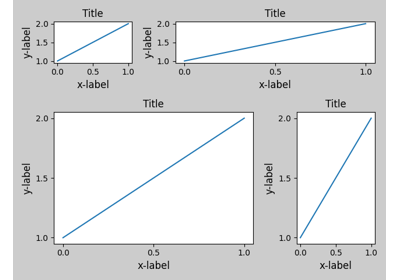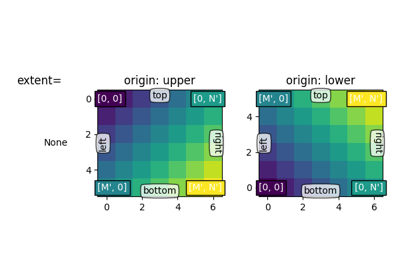# matplotlib.gridspec.GridSpecBase¶

class matplotlib.gridspec.GridSpecBase(nrows, ncols, height_ratios=None, width_ratios=None)[source]

Bases: object

A base class of GridSpec that specifies the geometry of the grid that a subplot will be placed.

Parameters: nrows, ncolsintThe number of rows and columns of the grid. width_ratiosarray-like of length ncols, optionalDefines the relative widths of the columns. Each column gets a relative width of width_ratios[i] / sum(width_ratios). If not given, all columns will have the same width. height_ratiosarray-like of length nrows, optionalDefines the relative heights of the rows. Each column gets a relative height of height_ratios[i] / sum(height_ratios). If not given, all rows will have the same height.
get_geometry(self)[source]

Return a tuple containing the number of rows and columns in the grid.

get_grid_positions(self, fig, raw=False)[source]

Return the positions of the grid cells in figure coordinates.

Parameters: figFigureThe figure the grid should be applied to. The subplot parameters (margins and spacing between subplots) are taken from fig. rawbool, default: FalseIf True, the subplot parameters of the figure are not taken into account. The grid spans the range [0, 1] in both directions without margins and there is no space between grid cells. This is used for constrained_layout. bottoms, tops, lefts, rightsarrayThe bottom, top, left, right positions of the grid cells in figure coordinates.
get_height_ratios(self)[source]

Return the height ratios.

This is None if no height ratios have been set explicitly.

get_subplot_params(self, figure=None)[source]
get_width_ratios(self)[source]

Return the width ratios.

This is None if no width ratios have been set explicitly.

property ncols

The number of columns in the grid.

new_subplotspec(self, loc, rowspan=1, colspan=1)[source]

Create and return a SubplotSpec instance.

Parameters: loc(int, int)The position of the subplot in the grid as (row_index, column_index). rowspan, colspanint, default: 1The number of rows and columns the subplot should span in the grid.
property nrows

The number of rows in the grid.

set_height_ratios(self, height_ratios)[source]

Set the relative heights of the rows.

height_ratios must be of length nrows. Each row gets a relative height of height_ratios[i] / sum(height_ratios).

set_width_ratios(self, width_ratios)[source]

Set the relative widths of the columns.

width_ratios must be of length ncols. Each column gets a relative width of width_ratios[i] / sum(width_ratios).

## Examples using matplotlib.gridspec.GridSpecBase¶